# 开发者实战 | OpenVINO™ 赋能 BLIP 实现视觉语言 AI 边缘部署

09/04 17:00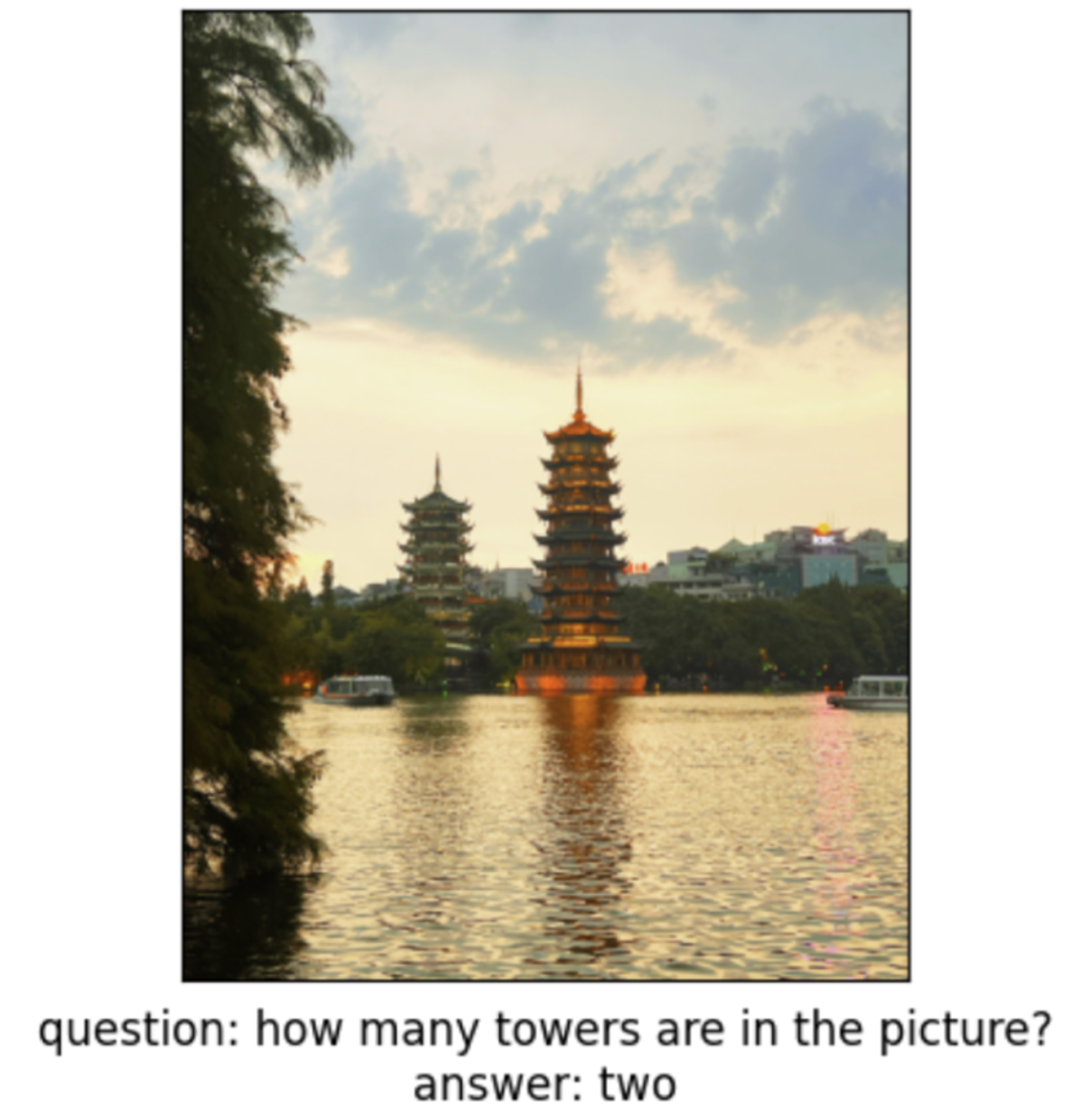BLIP 的预训练过程涉及两个关键组件：图像编码器和文本编码器。图像编码器负责将输入的图像转换为低维向量表示，而文本编码器则将输入的文本转换为另一个低维向量表示。为了实现统一的视觉-语言预训练，BLIP 采用了一种跨模态约束策略，即在预训练阶段，图像编码器和文本编码器被设计成相互约束的。这样的约束机制强制模型学习将视觉信息和语言信息进行对齐，从而使得模型在后续任务中能够更好地处理视觉与语言之间的联合信息。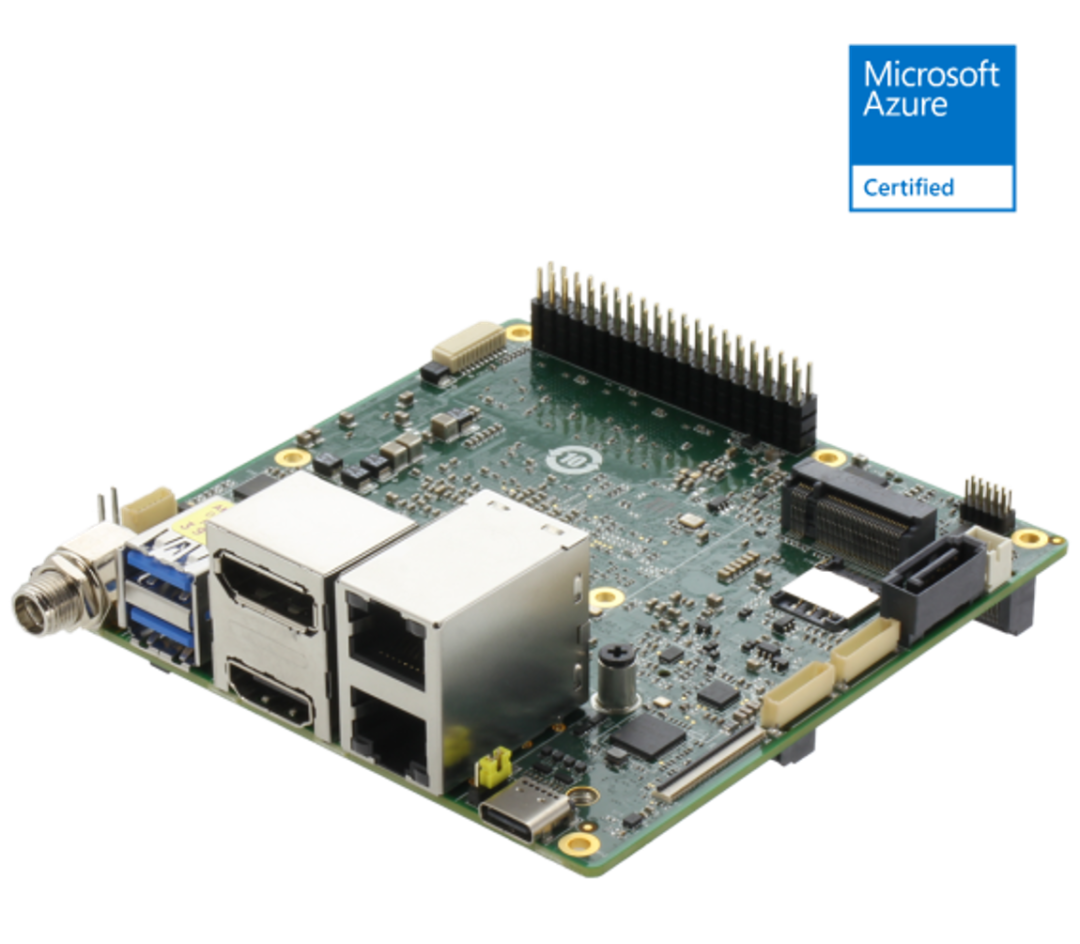1.4 倍以上 CPU 性能提升

UP Squared Pro 7000 采用 Intel® Core™/Atom®/N- 系列处理器，CPU 性能是上一代的 1.4 倍。UP Squared Pro 7000 拥有多达 8 个 Gracemont 内核，支持 OpenVINO™ Toolkit，以及第 12 代 Intel® 处理器的 UHD 显卡，拥有强大的计算能力、优化的推理引擎和图像处理功能，提供绝佳的智能解决方案。

UP Squared Pro 7000 配备 HDMI 2.0b、DP 1.2 埠和透过 USB Type-C 的 DP 1.4a，拥有出色的显示接口。UP Squared Pro 7000 整合了 GPU 和多重输出，可以同步支持三个 4K 显示器，非常适合用于数字广告牌等视觉导向型的相关应用。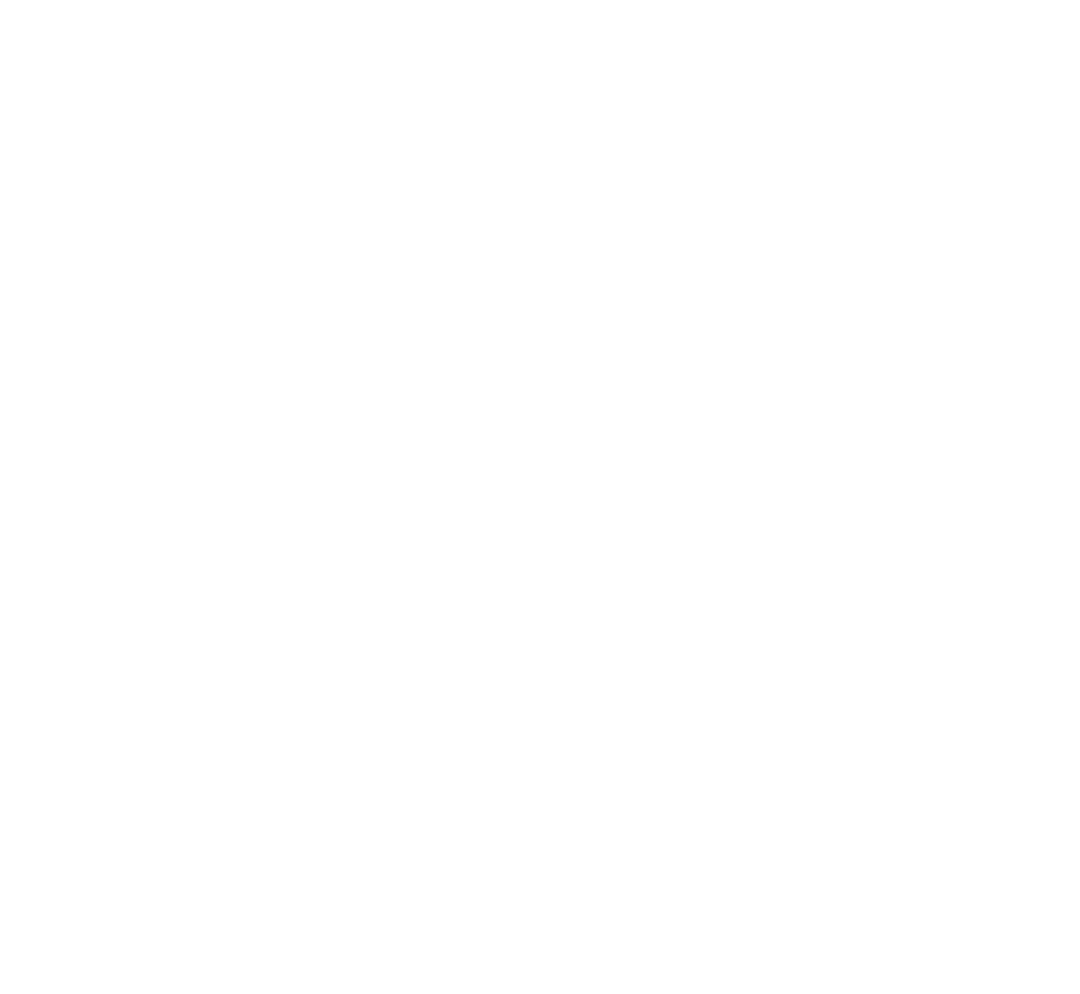!pip install "transformers >= 4.26.0"

import sysimport timefrom PIL import Imagefrom transformers import BlipProcessor, BlipForQuestionAnsweringsys.path.append("../utils")from notebook_utils import download_file# Get model and processorprocessor = BlipProcessor.from_pretrained("Salesforce/blip-vqa-base")model = BlipForQuestionAnswering.from_pretrained("Salesforce/blip-vqa-base")TEXT_ENCODER_OV = Path("blip_text_encoder.xml")TEXT_ENCODER_ONNX = TEXT_ENCODER_OV.with_suffix(".onnx")text_encoder = model.text_encodertext_encoder.eval()# if openvino model does not exist, convert it to onnx and then to IRif not TEXT_ENCODER_OV.exists():    if not TEXT_ENCODER_ONNX.exists():        # prepare example inputs for ONNX export        image_embeds = vision_outputs        image_attention_mask = torch.ones(image_embeds.size()[:-1], dtype=torch.long)        input_dict = {"input_ids": inputs["input_ids"], "attention_mask": inputs["attention_mask"], "encoder_hidden_states": image_embeds, "encoder_attention_mask": image_attention_mask}        # specify variable length axes        dynamic_axes = {"input_ids": {1: "seq_len"}, "attention_mask": {1: "seq_len"}}        # export PyTorch model to ONNX        with torch.no_grad():            torch.onnx.export(text_encoder, input_dict, TEXT_ENCODER_ONNX, input_names=list(input_dict), dynamic_axes=dynamic_axes)    # convert ONNX model to IR using model conversion Python API, use compress_to_fp16=True for compressing model weights to FP16 precision    ov_text_encoder = mo.convert_model(TEXT_ENCODER_ONNX, compress_to_fp16=True)    # save model on disk for next usages    serialize(ov_text_encoder, str(TEXT_ENCODER_OV))    print(f"Text encoder successfuly converted and saved to {TEXT_ENCODER_OV}")else:    print(f"Text encoder will be loaded from {TEXT_ENCODER_OV}")

text_decoder = model.text_decodertext_decoder.eval()TEXT_DECODER_OV = Path("blip_text_decoder.xml")TEXT_DECODER_ONNX = TEXT_DECODER_OV.with_suffix(".onnx")# prepare example inputs for ONNX exportinput_ids = torch.tensor([])  # begin of sequence token idattention_mask = torch.tensor([])  # attention mask for input_idsencoder_hidden_states = torch.rand((1, 10, 768))  # encoder last hidden state from text_encoderencoder_attention_mask = torch.ones((1, 10), dtype=torch.long)  # attention mask for encoder hidden statesinput_dict = {"input_ids": input_ids, "attention_mask": attention_mask, "encoder_hidden_states": encoder_hidden_states, "encoder_attention_mask": encoder_attention_mask}# specify variable length axesdynamic_axes = {"input_ids": {1: "seq_len"}, "attention_mask": {1: "seq_len"}, "encoder_hidden_states": {1: "enc_seq_len"}, "encoder_attention_mask": {1: "enc_seq_len"}}# specify output names, logits is main output of modeloutput_names = ["logits"]# past key values outputs are output for caching model hidden statepast_key_values_outs = []text_decoder_outs = text_decoder(**input_dict)for idx, _ in enumerate(text_decoder_outs["past_key_values"]):    past_key_values_outs.extend([f"out_past_key_value.{idx}.key", f"out_past_key_value.{idx}.value"])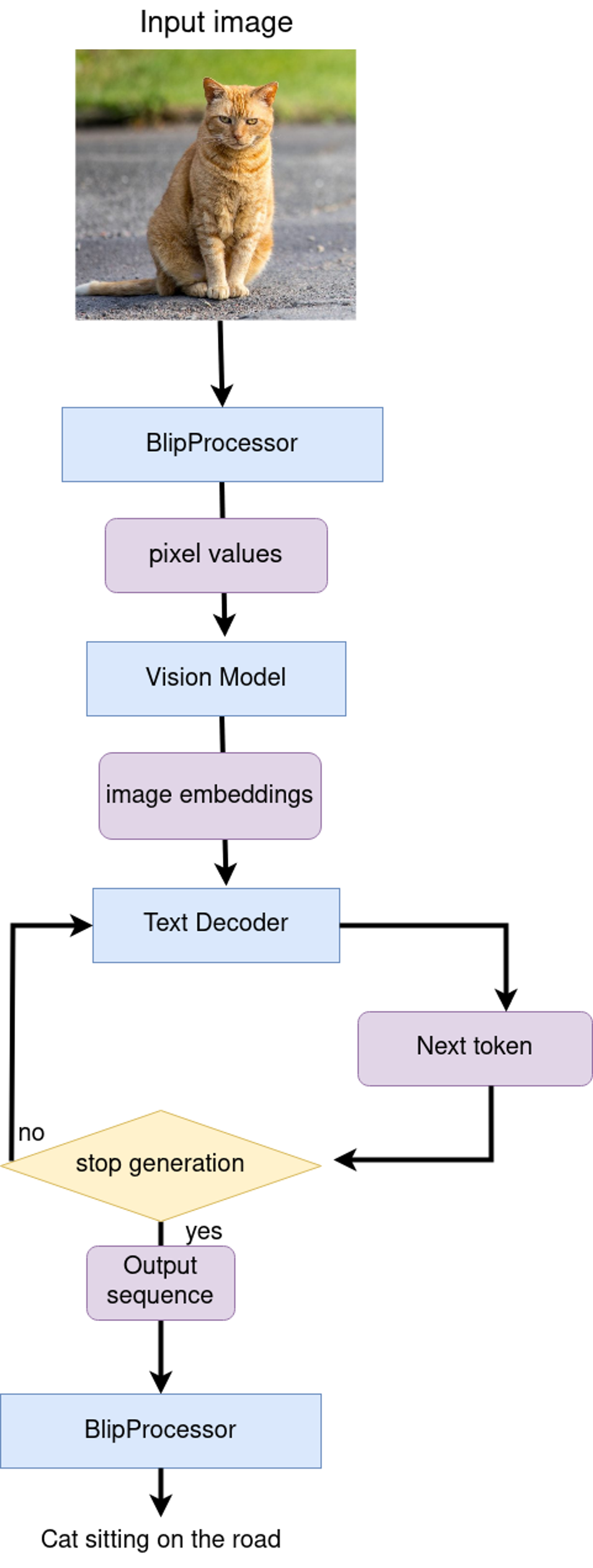class OVBlipModel:    """     Model class for inference BLIP model with OpenVINO    """    def __init__(self, config, decoder_start_token_id:int, vision_model, text_encoder, text_decoder):        """        Initialization class parameters        """        self.vision_model = vision_model        self.vision_model_out = vision_model.output(0)        self.text_encoder = text_encoder        self.text_encoder_out = text_encoder.output(0)        self.text_decoder = text_decoder        self.config = config        self.decoder_start_token_id = decoder_start_token_id        self.decoder_input_ids = c

    def generate_caption(self, pixel_values:torch.Tensor, input_ids:torch.Tensor = None, attention_mask:torch.Tensor = None, **generate_kwargs):        """        Image Captioning prediction        Parameters:          pixel_values (torch.Tensor): preprocessed image pixel values          input_ids (torch.Tensor, *optional*, None): pregenerated caption token ids after tokenization, if provided caption generation continue provided text          attention_mask (torch.Tensor): attention mask for caption tokens, used only if input_ids provided        Retruns:          generation output (torch.Tensor): tensor which represents sequence of generated caption token ids        """        batch_size = pixel_values.shape        image_embeds = self.vision_model(pixel_values.detach().numpy())[self.vision_model_out]        image_attention_mask = torch.ones(image_embeds.shape[:-1], dtype=torch.long)        if isinstance(input_ids, list):            input_ids = torch.LongTensor(input_ids)        elif input_ids is None:            input_ids = (                torch.LongTensor([[self.config.text_config.bos_token_id, self.config.text_config.eos_token_id]])                .repeat(batch_size, 1)            )        input_ids[:, 0] = self.config.text_config.bos_token_id        attention_mask = attention_mask[:, :-1] if attention_mask is not None else None        outputs = self.text_decoder.generate(            input_ids=input_ids[:, :-1],            eos_token_id=self.config.text_config.sep_token_id,            pad_token_id=self.config.text_config.pad_token_id,            attention_mask=attention_mask,            encoder_hidden_states=torch.from_numpy(image_embeds),            encoder_attention_mask=image_attention_mask,            **generate_kwargs,        )        return outputs

     def generate_answer(self, pixel_values:torch.Tensor, input_ids:torch.Tensor, attention_mask:torch.Tensor, **generate_kwargs):        """        Visual Question Answering prediction        Parameters:          pixel_values (torch.Tensor): preprocessed image pixel values          input_ids (torch.Tensor): question token ids after tokenization          attention_mask (torch.Tensor): attention mask for question tokens        Retruns:          generation output (torch.Tensor): tensor which represents sequence of generated answer token ids        """        image_embed = self.vision_model(pixel_values.detach().numpy())[self.vision_model_out]        image_attention_mask = np.ones(image_embed.shape[:-1], dtype=int)        if isinstance(input_ids, list):            input_ids = torch.LongTensor(input_ids)        question_embeds = self.text_encoder([input_ids.detach().numpy(), attention_mask.detach().numpy(), image_embed, image_attention_mask])[self.text_encoder_out]        question_attention_mask = np.ones(question_embeds.shape[:-1], dtype=int)        bos_ids = np.full((question_embeds.shape, 1), fill_value=self.decoder_start_token_id)        outputs = self.text_decoder.generate(            input_ids=torch.from_numpy(bos_ids),            eos_token_id=self.config.text_config.sep_token_id,            pad_token_id=self.config.text_config.pad_token_id,            encoder_hidden_states=torch.from_numpy(question_embeds),            encoder_attention_mask=torch.from_numpy(question_attention_mask),            **generate_kwargs,        )        return outputs

# create OpenVINO Core object instancecore = Core()import ipywidgets as widgetsdevice = widgets.Dropdown(    options=core.available_devices + ["AUTO"],    value='AUTO',    description='Device:',    disabled=False,)device# load models on deviceov_vision_model = core.compile_model(VISION_MODEL_OV, device.value)ov_text_encoder = core.compile_model(TEXT_ENCODER_OV, device.value)ov_text_decoder = core.compile_model(TEXT_DECODER_OV, device.value)ov_text_decoder_with_past = core.compile_model(TEXT_DECODER_WITH_PAST_OV, device.value)

out = ov_model.generate_caption(inputs["pixel_values"], max_length=20)caption = processor.decode(out, skip_special_tokens=True)fig = visualize_results(raw_image, caption)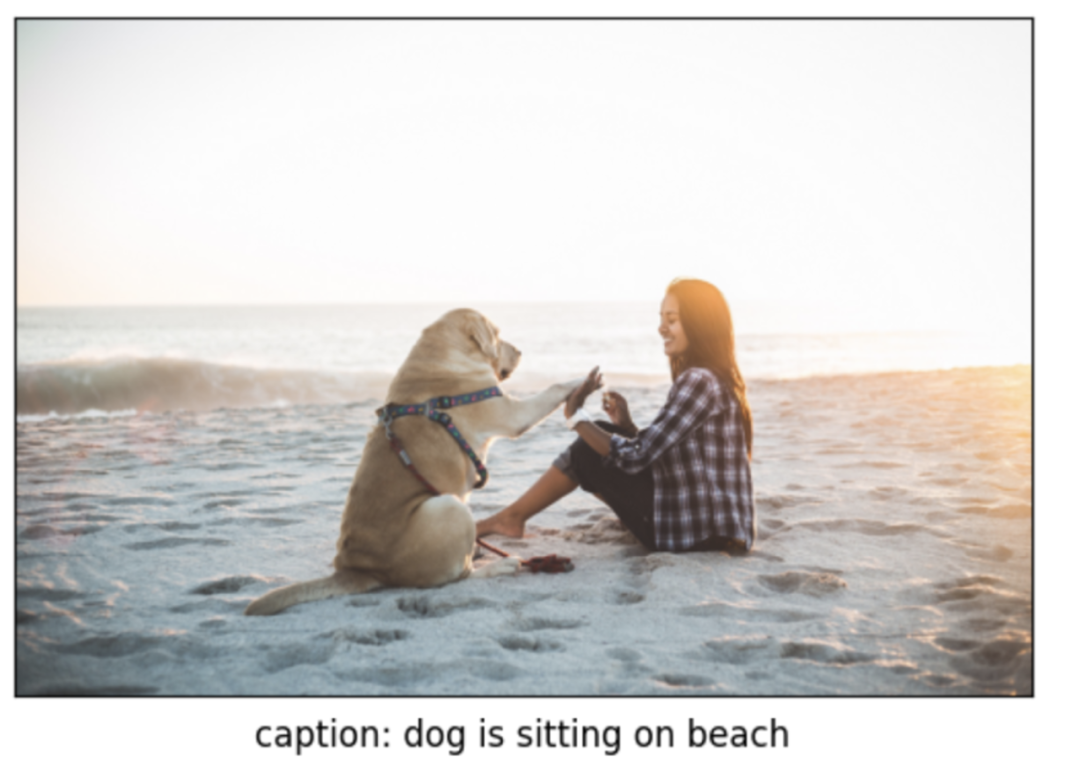start = time.perf_counter()out = ov_model.generate_answer(**inputs, max_length=20)end = time.perf_counter() - startanswer = processor.decode(out, skip_special_tokens=True)fig = visualize_results(raw_image, answer, question)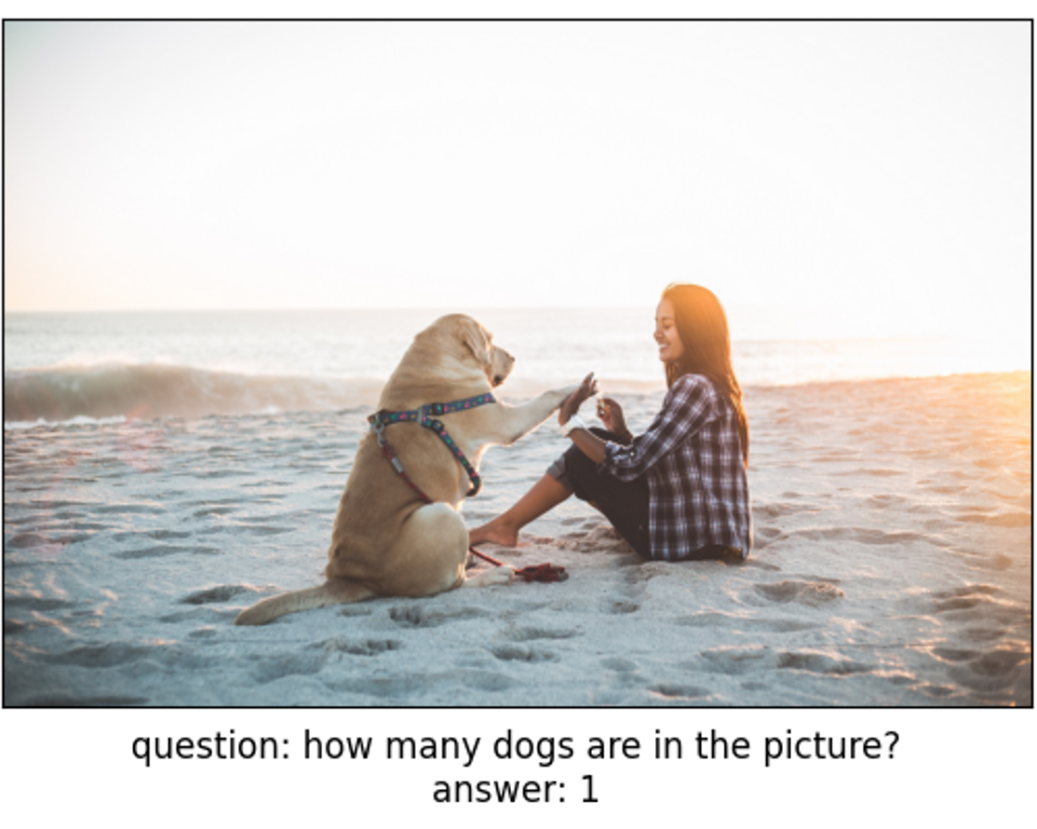Upsquared Pro 7000 系列:

https://ic-item.jd.com/10074763043245.html

 blip-vqa-base:

https://huggingface.co/Salesforce/blip-vqa-base

 notebooks:

https://github.com/openvinotoolkit/openvino_notebooks/tree/main/notebooks/233-blip-visual-language-processing

Intel技术可能需要启用硬件、软件或服务激活。

OpenVINO™

--END--



你也许想了解(点击蓝字查看)⬇️

➡️ 基于 Llama2 和 OpenVINO™ 打造聊天机器人

➡️ OpenVINO™ DevCon 2023重磅回归！英特尔以创新产品激发开发者无限潜能

➡️ 5周年更新 | OpenVINO™  2023.0，让AI部署和加速更容易

➡️ OpenVINO™5周年重头戏！2023.0版本持续升级AI部署和加速性能

➡️ OpenVINO™2023.0实战 | 在 LabVIEW 中部署 YOLOv8 目标检测模型

➡️ 开发者实战系列资源包来啦！

➡️

以AI作画，祝她节日快乐；简单三步，OpenVINO™ 助你轻松体验AIGC

➡️

还不知道如何用OpenVINO™作画？点击了解教程。

➡️

➡️

使用OpenVINO 在“端—边—云”快速实现高性能人工智能推理

➡️

图片提取文字很神奇？试试三步实现OCR！

➡️

【Notebook系列第六期】基于Pytorch预训练模型，实现语义分割任务

➡️

使用OpenVINO™ 预处理API进一步提升YOLOv5推理性能





扫描下方二维码立即体验
OpenVINO™ 工具套件 2023.0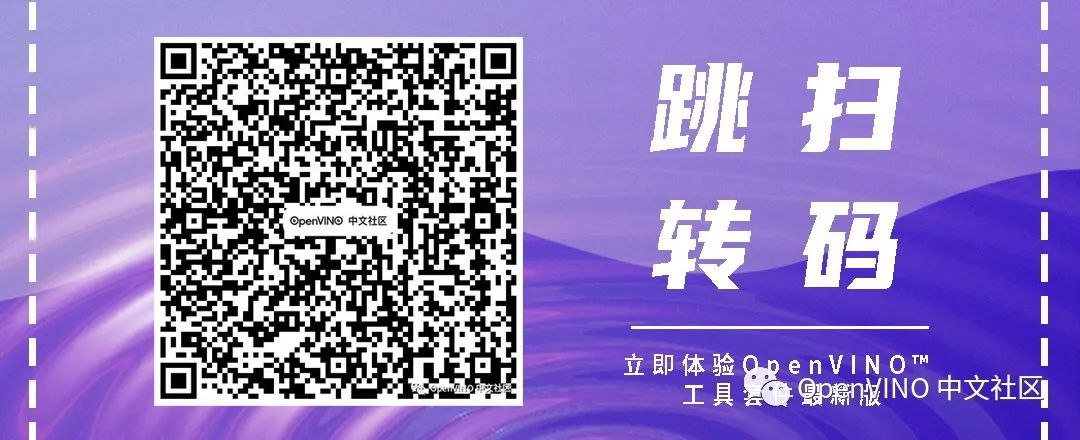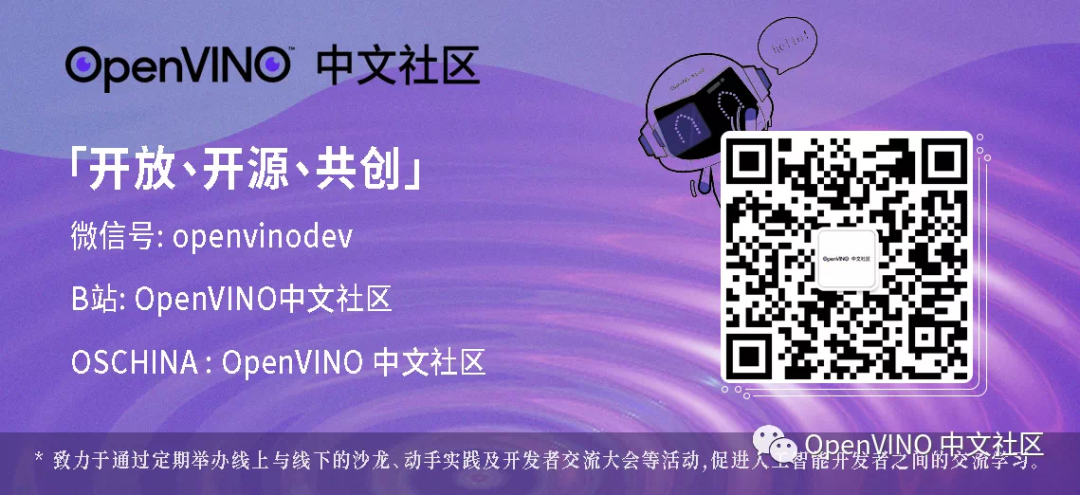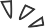0 评论
0 收藏
0